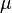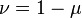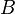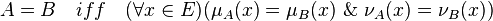16-17 May 2019 • Sofia, Bulgaria

Submission: 21 February 2019Notification: 11 March 2019Final Version: 1 April 2019

# Intuitionistic fuzzy sets

Intuitionistic fuzzy sets are sets whose elements have degrees of membership and non-membership. Intuitionistic fuzzy sets have been introduced by Krassimir Atanassov (1983) as an extension of Lotfi Zadeh's notion of fuzzy set, which itself extends the classical notion of a set.

• In classical set theory, the membership of elements in a set is assessed in binary terms according to a bivalent condition — an element either belongs or does not belong to the set.
• As an extension, fuzzy set theory permits the gradual assessment of the membership of elements in a set; this is described with the aid of a membership function valued in the real unit interval [0, 1].
• The theory of intuitionistic fuzzy sets further extends both concepts by allowing the assessment of the elements by two functions:$\mu$ for membership and$\nu$ for non-membership, which belong to the real unit interval [0, 1] and whose sum belongs to the same interval, as well.

Intuitionistic fuzzy sets generalize fuzzy sets, since the indicator functions of fuzzy sets are special cases of the membership and non-membership functions$\mu$ and$\nu$ of intuitionistic fuzzy sets, in the case when the strict equality exists:$\nu = 1 - \mu$, i.e. the non-membership function fully complements the membership function to 1, not leaving room for any uncertainty.

## Operations, relations, operators

For every two intuitionistic fuzzy sets$A$ and$B$ various relations and operations have been defined, most important of which are:

• Inclusion:$A \subset B \ \ \ iff \ \ \ (\forall x \in E)(\mu_A(x) \le \mu_B(x) \ \& \ \nu_A(x) \ge \nu_B(x))$ ,$A \supset B \ \ \ iff \ \ \ B \subset A$
• Equality:$A = B \ \ \ iff \ \ \ (\forall x \in E)(\mu_A(x) = \mu_B(x) \ \& \ \nu_A(x) = \nu_B(x))$
• Classical negation:$\overline{A} = \lbrace \langle x, \nu_A(x), \mu_A(x) \rangle \ | \ x \in E \rbrace$
• Conjuncion:$A \cap B = \lbrace \langle x, min(\mu_A(x), \mu_B(x)), max(\nu_A(x), \nu_B(X)) \rangle \ | \ x \in E \rbrace$
• Disjunction:$A \cup B = \lbrace \langle x, max(\mu_A(x), \mu_B(x)), min(\nu_A(x), \nu_B(X)) \rangle \ | \ x \in E \rbrace$

These operations and relations are defined similarly to these from the fuzzy set theory. More interesting are the modal operators that can be defined over intuitionistic fuzzy sets. These have no analogue in fuzzy set theory.